# Gaussian integral using Feynman’s technique

In my last post we evaluated the following definite integral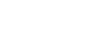This is the formula we got: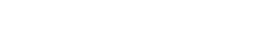and this is the integral we want to evaluate: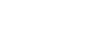which is equivalent to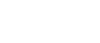because of symmetry: this is an even function, and since the integral has bounds –a and a, it becomes what I’ve just shown.

Here is the graph:

Since we have the formula, we can simply plug in the 2 and, voilà, we have the answer! We are going to do all of the calculations in a moment, but let’s actually substitute the 2 to see what the result is;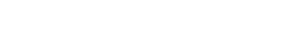If you type 0.5! on Google you’ll get 0.88622692545 , which is the square root of pi. That’s a very beautiful result in my opinion!

But now, let’s actually find the result using Feynman’s technique, or differentiation under the integral sign. I talk about it in this post, where I show how to evaluate the integral from 0 to infinity of sin(x)/x. I am going to use the same approach I used in my previous post, for the generalised form of this integral.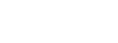an u-substitution would be enough; in order to get that extra x we need to write a new function with a parameter so that, when differentiating with respect to that parameter, the x we need will be generated.

We are going to write the integral as follows: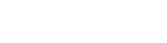this means that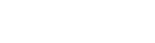we will need this later on, but for now, let’s rewrite the parameterized integral:As we saw here, let’s take the derivative with respect to a on both sides: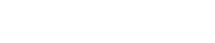The derivative can be brought inside the integral, but it becomes partial derivative, since it’s the derivative of each term of the sum; it works the same way as a regular derivative though: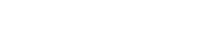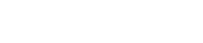Now we can perform a u-substitution: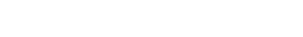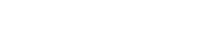Don’t forget about the bounds of integration: u=ax², therefore the lower bound is u₁=ax₁²=0 and the upper bound is u₂=ax₂²=infinity. In this case they don’t change, but always remember to do this.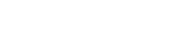Since we are integrating with respect to u, we need to write the x in terms of u. We know that u=ax²2, therefore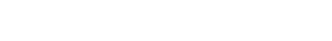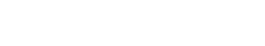This is the gamma function! Hence,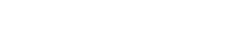or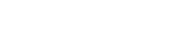Now we can take the integral on both sides, since this is I’(a);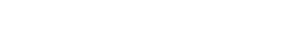Let’s apply linearity: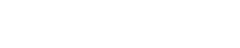Let’s now apply the power rule: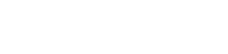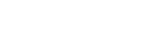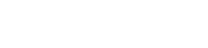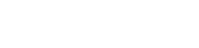Don’t forget the +C!

Overall, we can write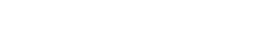Now, we need to find a number that makes the integral equal to 0 in order to find C; after that we will plug in the 1 that we had at the beginning in the original integral and we will find the answer. When a=infinity, we get e^-infinity, which is equal to 0, and therefore the whole integral: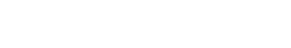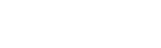When infinity is raised to a negative number, it gives 0; this is why: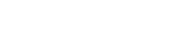Anyway,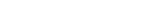Now, if you remember, we know that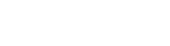when a=1; therefore, to find its value, we will plug 1 into this: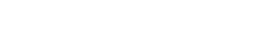Hence,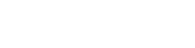We now know that 0.5!=sqrt(pi)/2, so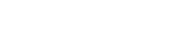Finally,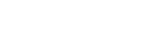And we’re done!

Hope you liked this post, and if you did give it a like and subscribe to stay updated, it would mean so much to me! If you have any doubts, questions, suggestions, leave a comment and I’ll be happy to reply!

Join 31 other subscribers.